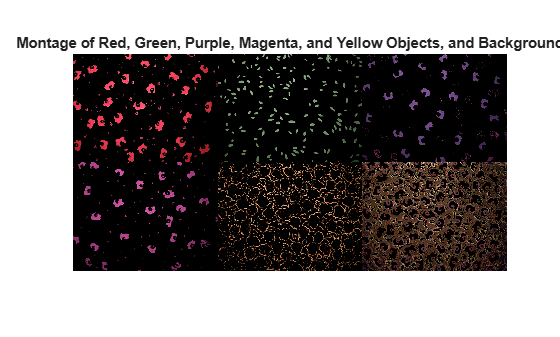# Color-Based Segmentation Using the L*a*b* Color Space

This example shows how to identify different colors in fabric by analyzing the L*a*b* colorspace.

### Step 1: Acquire Image

Read in the `fabric.png` image, which is an image of colorful fabric.

```fabric = imread("fabric.png"); imshow(fabric) title("Fabric")```### Step 2: Calculate Sample Colors in L*a*b* Color Space for Each Region

You can see six major colors in the image: the background color, red, green, purple, yellow, and magenta. Notice how easily you can visually distinguish these colors from one another. The L*a*b* colorspace (also known as CIELAB or CIE L*a*b*) enables you to quantify these visual differences.

The L*a*b* color space is derived from the CIE XYZ tristimulus values. The L*a*b* space consists of a luminosity 'L*' or brightness layer, chromaticity layer 'a*' indicating where color falls along the red-green axis, and chromaticity layer 'b*' indicating where the color falls along the blue-yellow axis.

Your approach is to choose a small sample region for each color and to calculate each sample region's average color in 'a*b*' space. You will use these color markers to classify each pixel.

To simplify this example, load the region coordinates that are stored in a MAT-file.

```load regioncoordinates; nColors = 6; sample_regions = false([size(fabric,1) size(fabric,2) nColors]); for count = 1:nColors sample_regions(:,:,count) = roipoly(fabric,region_coordinates(:,1,count), ... region_coordinates(:,2,count)); end imshow(sample_regions(:,:,2)) title("Sample Region for Red")```Convert your fabric RGB image into an L*a*b* image using the `rgb2lab` function.

`lab_fabric = rgb2lab(fabric);`

Calculate the mean 'a*' and 'b*' value for each area that you extracted with `roipoly`. These values serve as your color markers in 'a*b*' space.

```a = lab_fabric(:,:,2); b = lab_fabric(:,:,3); color_markers = zeros([nColors, 2]); for count = 1:nColors color_markers(count,1) = mean2(a(sample_regions(:,:,count))); color_markers(count,2) = mean2(b(sample_regions(:,:,count))); end```

For example, the average color of the red sample region in a*b* space is

`disp([color_markers(2,1), color_markers(2,2)]);`
``` 69.8278 20.1056 ```

### Step 3: Classify Each Pixel Using the Nearest Neighbor Rule

Each color marker now has an a* and a b* value. You can classify each pixel in the `lab_fabric` image by calculating the Euclidean distance between that pixel and each color marker. The smallest distance will tell you that the pixel most closely matches that color marker. For example, if the distance between a pixel and the red color marker is the smallest, then the pixel would be labeled as a red pixel.

Create an array that contains your color labels: 0 = background, 1 = red, 2 = green, 3 = purple, 4 = magenta, and 5 = yellow.

`color_labels = 0:nColors-1;`

Initialize matrices to be used in the nearest neighbor classification.

```a = double(a); b = double(b); distance = zeros([size(a), nColors]);```

Perform classification

```for count = 1:nColors distance(:,:,count) = ( (a - color_markers(count,1)).^2 + ... (b - color_markers(count,2)).^2 ).^0.5; end [~,label] = min(distance,[],3); label = color_labels(label); clear distance;```

### Step 4: Display Results of Nearest Neighbor Classification

The label matrix contains a color label for each pixel in the fabric image. Use the label matrix to separate objects in the original fabric image by color.

```rgb_label = repmat(label,[1 1 3]); segmented_images = zeros([size(fabric), nColors],"uint8"); for count = 1:nColors color = fabric; color(rgb_label ~= color_labels(count)) = 0; segmented_images(:,:,:,count) = color; end ```

Display the five segmented colors as a montage. Also display the background pixels in the image that are not classified as a color.

```montage({segmented_images(:,:,:,2),segmented_images(:,:,:,3) ... segmented_images(:,:,:,4),segmented_images(:,:,:,5) ... segmented_images(:,:,:,6),segmented_images(:,:,:,1)}); title("Montage of Red, Green, Purple, Magenta, and Yellow Objects, and Background")```### Step 5: Display a* and b* Values of Labeled Colors

You can see how well the nearest neighbor classification separated the different color populations by plotting the a* and b* values of pixels that were classified into separate colors. For display purposes, label each point with its color label. Purple is not a named color value, so specify the color purple using a string with a hexadecimal color code.

```purple = "#774998"; plot_labels = ["k", "r", "g", purple, "m", "y"]; figure for count = 1:nColors plot_label = plot_labels(count); plot(a(label==count-1),b(label==count-1),".", ... MarkerEdgeColor=plot_label,MarkerFaceColor=plot_label); hold on end title("Scatterplot of Segmented Pixels in a*b* Space"); xlabel("a* Values"); ylabel("b* Values");```